## 6  Discrete and Hausdorff objects

Now we can begin to develop some general topology and logic in terms of the Euclidean and Phoa principles and monadicity.

For the remainder of the paper (apart from the last section), we shall work in a model (C,Σ) of the axioms in Remark 3.8, i.e. Σ is a Euclidean semilattice and the adjunction is monadic. The category could be Set, LKLoc, CDLatop, an elementary topos or a free category as considered in Theorem 4.2 and Remark 4.3. We shall also assume the dual Euclidean principle when discussing the dual concepts: closed subspaces, compact Hausdorff spaces and proper maps.

By Theorem 3.10, Σ classifies some class M of supports, which we call open inclusions. So far, M has been entirely abstract: the only maps that are obliged to belong to it are the isomorphisms. The diagonal map Δ:XX× X is always a split mono, so what happens if this is open or closed?

In accordance with our convention about Greek and italic letters (Remark 2.5), we use p0:X× YX and p1:X× YY instead of the more usual π for product projections, though we keep Δ for the diagonal.

Definition 6.1 An object XobC is said to be discrete if the diagonal XX× X is open.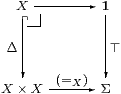The characteristic map (=X):X× X→Σ and its transpose { }X:X→ΣX are known as the equality predicate and singleton map respectively. We shall often write the subscript on this extensional (but internal) notion of equality, to distinguish it from the intensional (but external) equality of morphisms in the category C.

[Symbolically, the equality predicate =X is related to equality of morphisms by the rule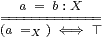in which a=b:X is called a statement in Definition I 4.4.]

Lemma 6.2 If X is discrete in this sense then it is T1, i.e. the Σ-order (Definition 1) on X is discrete. If all functions Σ→Σ are monotone then the link order is also discrete.

Proof    If xΣy then {x}⊑Σ{y} in ΣX by Remark 5.10(a), so, by putting φ≡evx in the definition of ⊑Σ, by reflexivity we have ⊤⇔ {x}(x)⊑{y}(x)≡(x=X y).

If all F:Σ→Σ are monotone then xL yxΣy. But for a direct argument (on the same hypothesis) consider F≡λσ.(ℓσ=Xℓ⊥). Then F⊥⇔ ⊤, so F⊤⇔ ⊤ by monotonicity, but this says that x=X y.         ▫

Examples 6.3

1. Every set is discrete.
2. For a poset to be discrete in this sense, the diagonal {(x,y) ∣ x=Xy} must be an upper subset of X× X. This means that if xy then (x,x)⊑(x,y) must also lie in this subset, so x=y. Hence discreteness agrees with standard usage. (This is the same argument as in the Lemma.)
3. For a topological space to be discrete, the diagonal subset must be open. Each singleton {x} is open, so if we may form arbitrary unions of open subsets, all subsets are open.
4. In recursion theory, ℕ is discrete in the sense of Definition 6.1 and singletons in ℕ are recursively enumerable, but arbitrary subsets are not. This is explored in [D]. Intuitionistically, even 1 has co-RE subspaces that are not RE, so there is nothing to be gained from introducing a notion of strong discreteness.
5. A presheaf is discrete with respect to Σ≡Ωj (Example 2) iff it is j-separated [Joh77, Proposition 3.29] [BW85, §6.2].
6. A recursive datatype X is discrete iff there is a program δ(x,y) that terminates iff its arguments are intensionally equal; for example if X is defined by reduction rules or by generators and relations then δ has to search for an equational proof. Following the usage of decidable (yes or no) and semi-decidable (yes or wait), semi-discrete would perhaps be a clearer term.

Definition 6.4 Dually, we say that an object is Hausdorff if the diagonal is closed (classified by ⊥) [Bou66, §8]. We write (≠X) or (#X):X× X→Σ for the characteristic function, which is sometimes called apartness. Again, it follows that singletons are closed (the T1 separation property in point-set topology), but not arbitrary subsets.

The symbolic rule isExercise 6.5 To check that you understand how ≠X is defined, adapt Lemma 6.2 to show that if xΣy in a Hausdorff space X then (xX y)⇔ ⊥, and explain how it follows from this that X is T1 in the order-theoretic sense.         ▫

Examples 6.6

1. For locales, this property is called strong Hausdorffness [Joh82, §III 1.3], but this is because localic and spatial products are not the same unless we require local compactness, as indeed we do in this paper.
2. An object of a topos is Hausdorff in our sense iff it is ¬¬-separated, and in particular this always happens for classical sets. Similarly, any discrete poset or space whose underlying set is ¬¬-separable is also Hausdorff, the converse also being true for posets. Hausdorffness is therefore not a very interesting property for sets and posets, and it is better to avoid this term altogether unless the dual Euclidean and Frobenius principles hold.
3. Following the analogy between open and recursively enumerable subsets, a recursive datatype X is Hausdorff iff there is a program δ(x,y) that terminates iff its arguments are unequal (distinguishable). For example, the real line ℝ is Hausdorff, but not discrete [Theorem I 9.3]. Of course, we know this topologically: the point is that this is the case computationally, as Brouwer tried to remind us, in contradiction to the pathological analysis that led to Cantor’s set theory, and “floating point” arithmetic in Fortran and other programming languages, which purport to make equality decidable.
4. In traditional point-set topology and locale theory, any T0 group is Hausdorff, as is any discrete space, but these results depend on being able to form arbitrary unions of open subsets, and are therefore not true recursively. For example, we would otherwise be able to solve the word problem for groups, i.e. detect that a group is non-trivial. In particular, even the singleton subgroup need not be closed.

To sum up, discreteness and Hausdorffness are quite different properties.

Lemma 6.7 Let X be discrete and φ∈ΣX. Then

 ∃Δ(φ) ≡ (λ x y.(x=X y)∧ φ x) ≡  (λ x y.(x=X y)∧ φ y).

Proof    We use the Frobenius law ∃Δ(θ∧ΣΔψ)≡ (∃Δθ)∧ψ, with θ≡⊤, so (∃Δθ)=(λ x y.x=X y). Putting either ψ≡Σp0φ or Σp1φ, so ψ=λ x y.φ(x) or λ x y.φ(y), we recover φ=ΣΔψ, so the left hand side of the Frobenius law is ∃Δ(φ) and the right hand side is one of the other two expressions.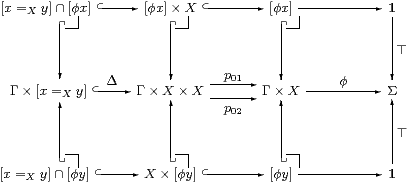Theorem 3.10 gives an alternative proof using open subsets: [(x=X y)∧ φ x] is the same subobject of Γ× X as [(x=X y)∧ φ y] since the composites XX× XX are equal; hence they are the also same subobject of Γ× X× X, so the characteristic maps are equal.         ▫

Corollary 6.8 (=X) is reflexive, symmetric and transitive.

Proof    Consider φ≡λ u.(y=X u) and φ≡λ u.(u=X z).         ▫

This is the algebraic characterisation of the equality predicate, which we consider in Section 10.

Corollary 6.9 n.φ(n)) a  ≡  ∃ n.(n=a)∧φ(n).

Here “∃ n” is as in Definition 7.7. So, instead of β-reducing the application of a predicate to an argument of type ℕ, i.e. substituting the term a for the variable n throughout the formula φ, we can make a local change to the expression-tree and rely on unification to carry out the effect of the substitution [Section A 11].         ▫

[Lemma J 5.9 proves the properties of equality symbolically from the Euclidean principle.]

Remark 6.10 The analogous property for ∀ in intuitionistic logic is that

 ∀Δ(φ)(x,y) ≡ (x=X y)⇒ φ x ≡  (x=X y)⇒ φ y.

We need the dual Euclidean and Frobenius principles (Corollary 5.6) to make this equivalent to the lattice dual of the Lemma, namely

 ∀Δ(φ)(x,y) ≡ (x≠X y ∨ φ x) ≡  (x≠X y∨ φ y),

for Hausdorff spaces. In this case, an object that is both discrete and Hausdorff is called decidable, cf. Proposition 9.6.

Proposition 6.11

1. 1 is discrete.
2. If Σ has ⊥ then 1 is also Hausdorff.
3. If X and Y are both discrete then so is X× Y.
4. Similarly if they are both Hausdorff, assuming that Σ is a distributive lattice.
5. If UX is any subset of (i.e. any mono into) a discrete or Hausdorff object then U is also discrete or Hausdorff.

Proof    [a,b] (=1)≡⊤:1×1→Σ and (≠1)≡⊥.
[c,d] (=X× Y)≡(=X)∧(=Y) and (≠X× Y)≡(≠X)∨(≠Y).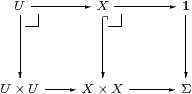[e] UX is mono iff the square on the left is a pullback.         ▫

The next result will be used to prove Theorem 11.3, so instead of monadicity we assume only that Σ is exponentiating, but still read the existence of the pullback in Definition 6.1 as the definition of discreteness.

Lemma 6.12 If X is discrete then the maps { }X:X→ΣX and ηX:X→ΣΣX are mono.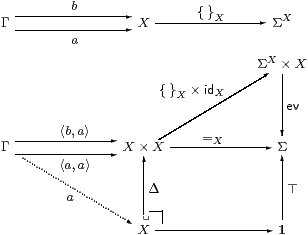Proof    If { }Xa={ }Xb then the composites Γ→Σ are equal, but one of them factors through the pullback, so the other does too.

Naturality of η with respect to { }X gives a commutative square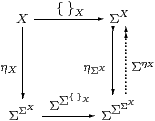The map on the right is split mono, so by the first part and cancellation, ηX is mono.         ▫

Remark 6.13 The Lemma helps to explain why there are two ways of defining j-separated presheaves (namely being T0 or discrete with respect to Ωj) and the fact that Hausdorffness implies sobriety for spaces [Joh82, Lemma II 1.6(ii)]. See also [Joh77, Definition 1.24], [BW85, §2.3, Proposition 6] and [Mik76, p. 3] concerning the singleton map { }X in an elementary topos.

We shall find at the end of the paper that CDLatop satisfies most of our characterisation of elementary toposes, apart from the fact that all sets are discrete. Let’s briefly explore this analogy.

Example 6.14 Assuming excluded middle, the subcategory SetPosCDLatop consists of the discrete (equivalently, Hausdorff) objects.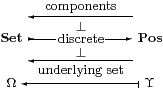Set is the reflective subcategory of the Ω-replete objects in Pos, just as the sheaf subtopos ℰj consists of the Ωj-replete objects in ℰ [BR98].

The object Ω (the dominance in Set) is the underlying set of Υ (that in Pos), i.e. its image under the right adjoint of the inclusion SetPos. The product-preserving left adjoint (components) to the inclusion of categories is the replete reflection.

By contrast, Ωj is the result of applying the left adjoint, sheafification, to Ω. Also, we have Ω↞Υ instead of Ωj⊂Ω.         ▫

Remarks 6.15 In the same way we may ask whether adjoints exist to the inclusions of the full subcategories Set and KHaus of (overt) discrete and compact Hausdorff spaces in LKLoc instead of Pos.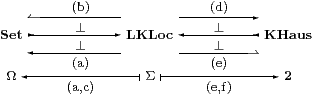1. The right adjoint SetLKLoc is the set of points functor.
2. Unfortunately, the left adjoint SetLKLoc, which is the components functor, is only defined for locally connected locales [BP80, Tay90], but it does preserve products.
3. The underlying set of the Sierpiński space Σ is the subobject classifier Ω, and the objects of the smaller category are replete , overt and discrete with respect to both Σ and Ω.
4. The left adjoint LKLocKHaus is the Stone–vCech compactification [Joh82, Theorem IV 2.1], but it does not preserve finite products.
5. Martín Escardó has shown that the patch topology provides the right adjoint, but only to the inclusion into the category of stably locally compact locales and perfect maps [Esc99].
6. The patch topology on the Sierpiński space is 2, but 2-replete objects are Stone spaces (totally disconnected compact Hausdorff spaces), and these do not form a pretopos.

The lack of open–closed symmetry between these results makes it very unlikely that they have a unifying formulation in our axiomatisation.

[The overt discrete objects play the role of sets in ASD. If we assume, motivated by (a) above, that the inclusion of the full subcategory of them has a right adjoint U, then this subcategory is a topos with Ω≡UΣ and the whole category is equivalent to that of locally compact locales over this topos, [H].]

Discreteness and Hausdorffness are binary properties, relating X to X× X: we now turn to the corresponding nullary ones, involving 1=X0.